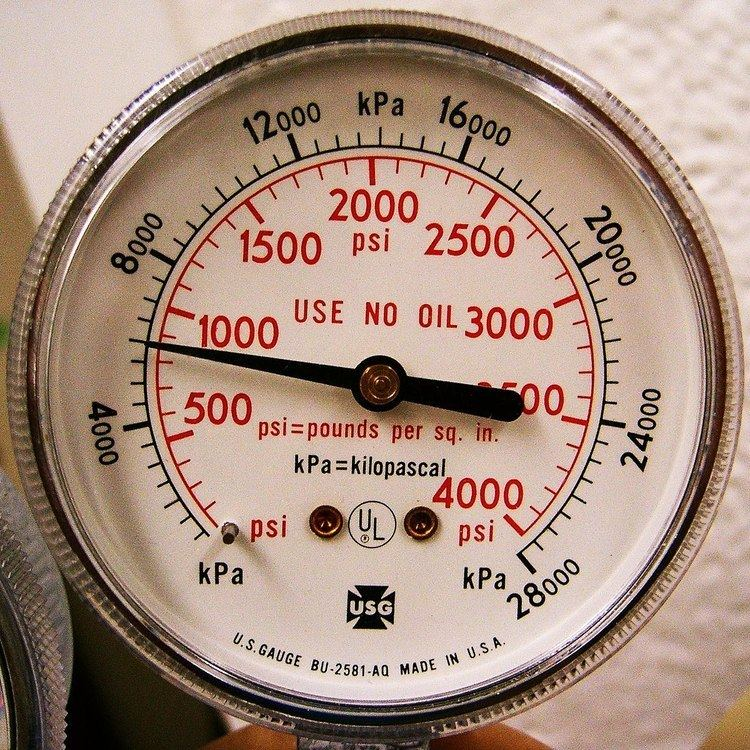# Pascal (unit)

Updated on
Edit
Like
Comment
 Unit system  SI derived unitSymbol  Pa1 Pa in ...  ... is equal to ... Unit of  Pressure or stressNamed after  Blaise PascalSI base units:  kg⋅m⋅sThe pascal (symbol: Pa) is the SI derived unit of pressure used to quantify internal pressure, stress, Young's modulus and ultimate tensile strength. It is defined as one newton per square meter. It is named after the French polymath Blaise Pascal.

## Contents

Common multiple units of the pascal are the hectopascal (1 hPa = 100 Pa) which is equal to one millibar.

The unit of measurement called standard atmosphere (atm) is defined as 101,325 Pa and approximates to the average pressure at sea-level at the latitude 45° N. Meteorological reports typically state atmospheric pressure in hectopascals.

## Etymology

The unit is named after Blaise Pascal, noted for his contributions to hydrodynamics and hydrostatics, and experiments with a barometer. The name pascal was adopted for the SI unit newton per square metre (N/m2) by the 14th General Conference on Weights and Measures in 1971.

## Definition

The pascal can be expressed using SI derived units, or alternatively solely SI base units, as:

1   P a = 1   N m 2 = 1   k g m s 2

where N is the newton, m is the metre, kg is the kilogram, and s is the second.

One pascal is the pressure exerted by a force of magnitude one newton perpendicularly upon an area of one square metre.

## Miscellaneous

The unit of measurement called atmosphere or standard atmosphere (atm) is 101325 Pa (101.325 kPa). This value is often used as a reference pressure and specified as such in some national and international standards, such as ISO 2787 (pneumatic tools and compressors), ISO 2533 (aerospace) and ISO 5024 (petroleum). In contrast, IUPAC recommends the use of 100 kPa as a standard pressure when reporting the properties of substances.

The Unicode computer character set has dedicated symbols U+33A9 Square Pa and U+33AA Square kPa in the CJK Compatibility block, but these exist for backward-compatibility with some older ideographic character-sets and are therefore deprecated.

## Uses

The pascal (Pa) or kilopascal (kPa) as a unit of pressure measurement is widely used throughout the world and has largely replaced the pounds per square inch (psi) unit, except in some countries that still use the imperial measurement system or the US customary system, including the United States.

Geophysicists use the gigapascal (GPa) in measuring or calculating tectonic stresses and pressures within the Earth.

Medical elastography measures tissue stiffness non-invasively with ultrasound or magnetic resonance imaging, and often displays the Young's modulus or shear modulus of tissue in kilopascals.

In materials science and engineering, the pascal measures the stiffness, tensile strength and compressive strength of materials. In engineering use, because the pascal represents a very small quantity, the megapascal (MPa) is the preferred unit for these uses.

The pascal is also equivalent to the SI unit of energy density, J/m3. This applies not only to the thermodynamics of pressurised gases, but also to the energy density of electric, magnetic, and gravitational fields.

In measurements of sound pressure, or loudness of sound, one pascal is equal to 94 decibels SPL. The quietest sound a human can hear, known as the threshold of hearing, is 0 dB SPL, or 20 µPa.

The airtightness of buildings is measured at 50 Pa.

## Hectopascal and millibar units

The units of atmospheric pressure commonly used in meteorology were formerly the bar, which was close to the average air pressure on Earth, and the millibar. Since the introduction of SI units, meteorologists generally measure pressures in hectopascals (hPa) unit, equal to 100 pascals or 1 millibar. Exceptions include Canada and Portugal, which use kilopascals (kPa). In many other fields of science, the SI is preferred, which means Pa with a prefix (in multiples of 1000) is preferred.

Many countries also use the millibar or hectopascal to give aviation altimeter settings. In practically all other fields, the kilopascal (1000 pascals) is used instead.

Pascal (unit) Wikipedia